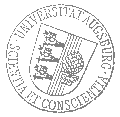# Universität AugsburgInstitut für MathematikOberseminar Differentialgeometrie

Prof. Em. Dr. Matthias Brack
Universität Regensburg

spricht am

Montag, 19. Juni 2023

um

16 Uhr s.t.

im

2004/L1 und Zoom (hybride Veranstaltung)

über das Thema:

## »Semiclassical trace formulae and distinct square partitions«

 Abstract: I will give a brief overview of the use of semiclassical trace formulae for the description of quantum spectra and of energy (or mass) oscillations in finite fermionic systems (such as atomic nuclei or metallic clusters). Then, I report on an application in Number Theory: to the partition P(n) of an integer n into sums of distinct squares. We obtain both the smooth part of P(n) and its oscillating part in the asymptotic limit of large n. For the latter, we have derived a semi-analytical trace formula in which the frequencies of the leading 'periodic orbits' are found amongst the smallest Pythagorean triplets. For n \simg 100'000, one 'orbit' reproduces the oscillations in P(n) exactly. Even for n as small as \sim 500, ten (classes of) 'orbits' are sufficient to reproduce the oscillations very accurately. If you want to take part via Zoom please ask Prof. Frauenfelder for­ login details: Urs.Frauenfelder@math.uni-augsburg.de

 Hierzu ergeht herzliche Einladung. Prof. Dr. Urs Frauenfelder

Kaffee, Tee und Gebäck eine halbe Stunde vor Vortragsbeginn im Raum 2006 (L1).

[Impressum]      [Datenschutz]      wwwadm@math.uni-augsburg.de,     Do 11-Mai-2023 11:06:40 MESZ